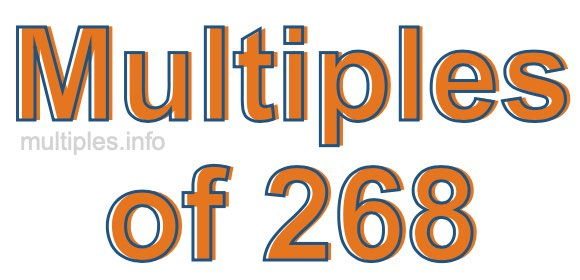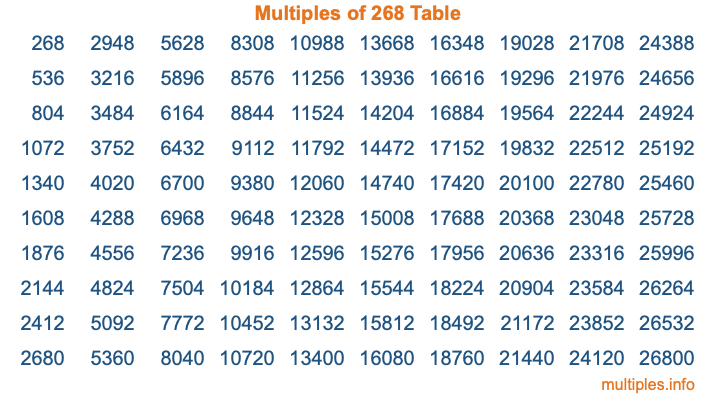Multiples of 268Welcome to the Multiples of 268 page. Here we will first teach you everything you will ever need to know about the multiples of 268, and then give you a study guide summary of everything we taught you to make sure you remember it all. Use this page to look up facts and learn information about the multiples of 268. This page will make you a multiples of two hundred sixty-eight expert!

Definition of Multiples of 268
Multiples of 268 are all the numbers that when divided by 268 equal an integer. Each of the multiples of 268 are called a multiple. A multiple of 268 is created by multiplying 268 by an integer.

Therefore, to create a list of multiples of 268, you start with 1 multiplied by 268, then 2 multiplied by 268, then 3 multiplied by 268, and so on for as long as you want. Thus, the list of the first five multiples of 268 is 268, 536, 804, 1072, and 1340. To see a larger list of multiples of 268, see the printable image of Multiples of 268 further down on this page. We also have a category where you can choose any nth multiple of 268.

Multiples of 268 Checker
The Multiples of 268 Checker below checks to see if any number of your choice is a multiple of 268. In other words, it checks to see if there is any number (integer) that when multiplied by 268 will equal your number. To do that, we divide your number by 268. If the the quotient is an integer, then your number is a multiple of 268.

Is  a multiple of 268?

Least Common Multiple of 268 and ...
A Least Common Multiple (LCM) is the lowest multiple that two or more numbers have in common. This is also called the smallest common multiple or lowest common multiple and is useful to know when you are adding our subtracting fractions. Enter one or more numbers below (268 is already entered) to find the LCM.

Check out our LCM Calculator if you need more details about the Least Common Multiple or if you need the LCM for different numbers for adding and subtraction fractions.

nth Multiple of 268
As we stated above, 268 is the first multiple of 268, 536 is the second multiple of 268, 804 is the third multiple of 268, and so on. Enter a number below to find the nth multiple of 268.

th multiple of 268

Multiples of 268 vs Factors of 268
268 is a multiple of 268 and a factor of 268, but that is where the similarities end. All postive multiples of 268 are 268 or greater than 268. All positive factors of 268 are 268 or less than 268.

Below is the beginning list of multiples of 268 and the factors of 268 so you can compare:

Multiples of 268: 268, 536, 804, 1072, 1340, etc.

Factors of 268: 1, 2, 4, 67, 134, 268

As you can see, the multiples of 268 are all the numbers that you can divide by 268 to get a whole number. The factors of 268, on the other hand, are all the whole numbers that you can multiply by another whole number to get 268.

It's also interesting to note that if a number (x) is a factor of 268, then 268 will also be a multiple of that number (x).

Multiples of 268 vs Divisors of 268
The divisors of 268 are all the integers that 268 can be divided by evenly. Below is a list of the divisors of 268.

Divisors of 268: 1, 2, 4, 67, 134, 268

The interesting thing to note here is that if you take any multiple of 268 and divide it by a divisor of 268, you will see that the quotient is an integer.

Multiples of 268 Table
Below is an image of the first 100 multiples of 268 in a table. The table is in chronological order, column by column. The first column has the first ten multiples of 268, the second column has the next ten multiples of 268, and so on.The Multiples of 268 Table is also referred to as the 268 Times Table or Times Table of 268. You are welcome to print out our table for your studies.

Negative Multiples of 268
Although not often discussed or needed in math, it is worth mentioning that you can make a list of negative multiples of 268 by multiplying 268 by -1, then by -2, then by -3, and so on, to get the following list of negative multiples of 268:

-268, -536, -804, -1072, -1340, etc.

Multiples of 268 Summary
Below is a summary of important Multiples of 268 facts that we have discussed on this page. To retain the knowledge on this page, we recommend that you read through the summary and explain to yourself or a study partner why they hold true.

There are an infinite number of multiples of 268.

A multiple of 268 divided by 268 will equal a whole number.

268 divided by a factor of 268 equals a divisor of 268.

The nth multiple of 268 is n times 268.

The largest factor of 268 is equal to the first positive multiple of 268.

268 is a multiple of every factor of 268.

268 is a multiple of 268.

A multiple of 268 divided by a divisor of 268 equals an integer.

268 divided by a divisor of 268 equals a factor of 268.

Any integer times 268 will equal a multiple of 268.

Multiples of a Number
Here you can get the multiples of another number, all with the same attention to detail as we did for multiples of 268 on this page.

Multiples of
Multiples of 269
Did you find our page about multiples of two hundred sixty-eight educational? Do you want more knowledge? Check out the multiples of the next number on our list!